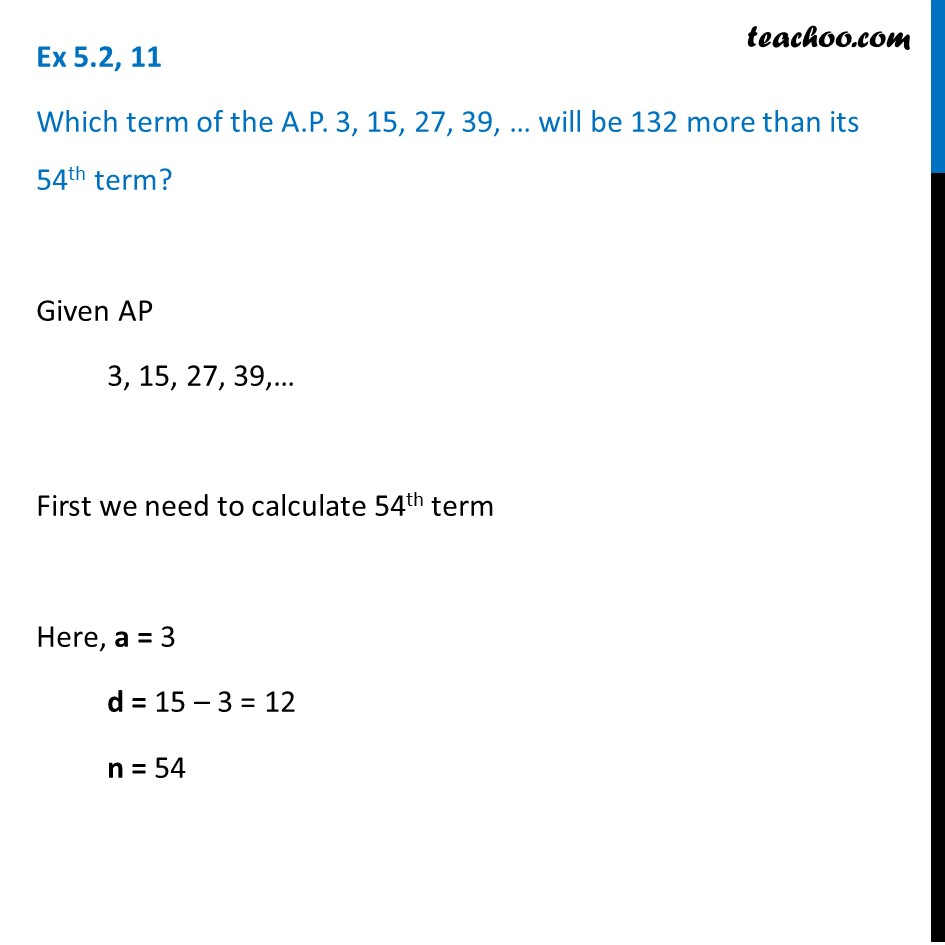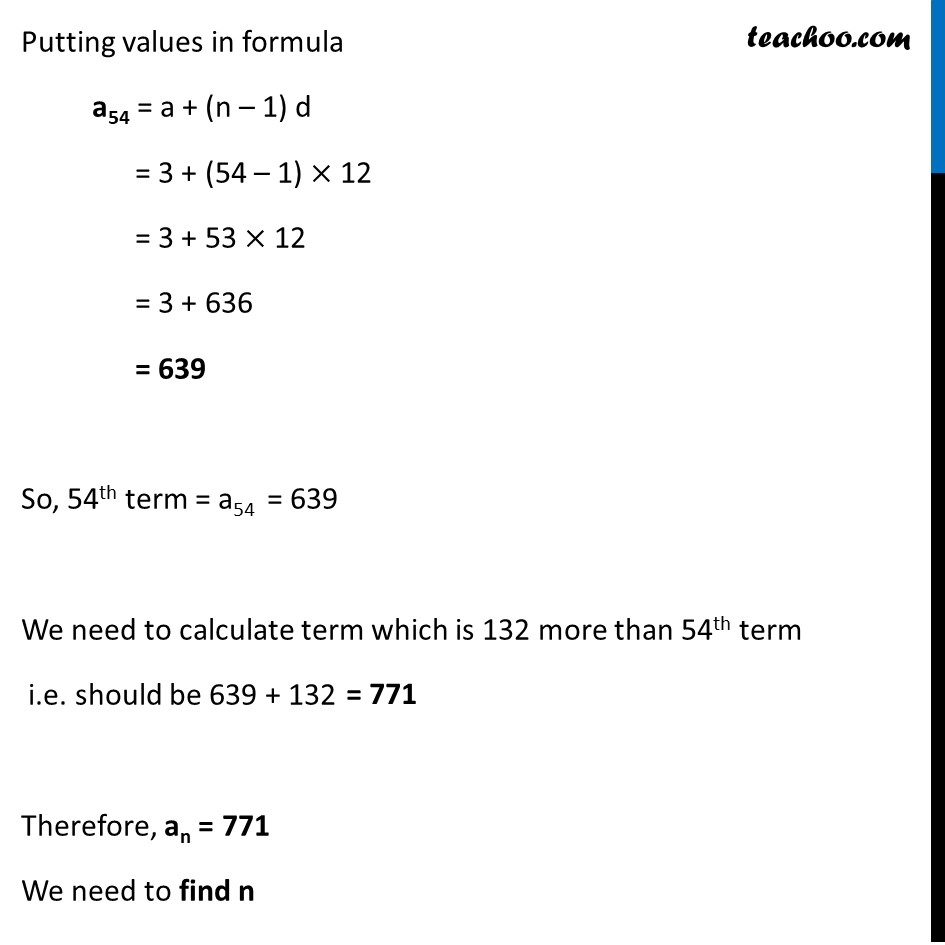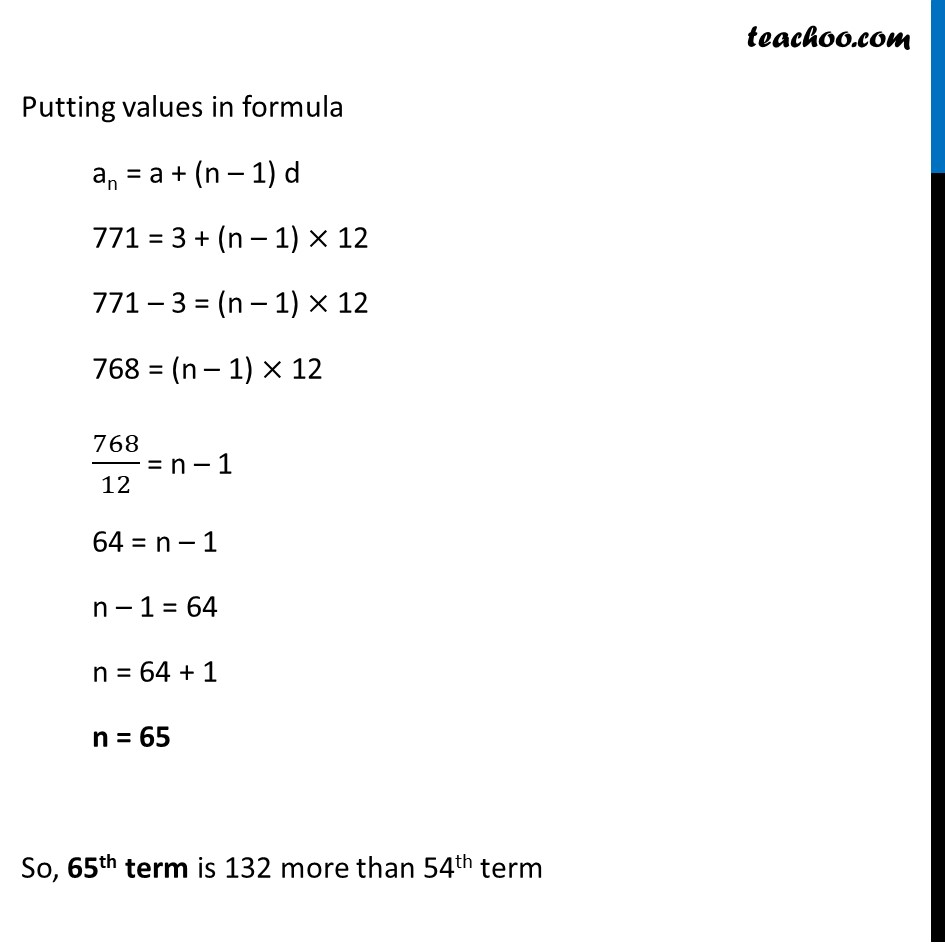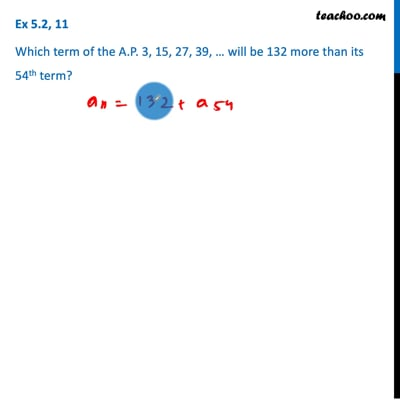Ex 5.2

Chapter 5 Class 10 Arithmetic Progressions (Term 2)
Serial order wiseThis video is only available for Teachoo black users

### Transcript

Ex 5.2, 11 Which term of the A.P. 3, 15, 27, 39, … will be 132 more than its 54th term? Given AP 3, 15, 27, 39,… First we need to calculate 54th term Here, a = 3 d = 15 – 3 = 12 n = 54 Putting values in formula a54 = a + (n – 1) d = 3 + (54 – 1) × 12 = 3 + 53 × 12 = 3 + 636 = 639 So, 54th term = a54 = 639 We need to calculate term which is 132 more than 54th term i.e. should be 639 + 132 Therefore, an = 771 We need to find n Putting values in formula an = a + (n – 1) d 771 = 3 + (n – 1) × 12 771 – 3 = (n – 1) × 12 768 = (n – 1) × 12 768/12 = n – 1 64 = n – 1 n – 1 = 64 n = 64 + 1 n = 65 So, 65th term is 132 more than 54th term